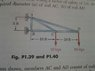# Solid Mechanics- Ultimate Load in truss.

ledphones

## Homework Statement

A 3/4-in.-diameter rod made of the same material as rods AC and AD in the truss shown was tested to failure and an ultimate load of 29 kips was recorded. Using a factor of safety of 3.0, determine the required diameter (a) of rod AC, (b) of rod AD.

## Homework Equations

τ(allowable)=τ(ultimate)/f.s.
A(req) = P/σ(allowable)
ƩM=0
ƩF(y)=0
ƩF(x)=0
Area=π/4*d^2

## The Attempt at a Solution

First I found the allowable load. 29/3= 9.66667

Next I took the moment about B finding the reaction of A in the x direction to be 60 kips.

By summing the forces in the x and y direction i found the reaction at B to be -60 and the reaction of A in the y to be 20.

This is where I go wrong. I take the force in rod AC to be √(20^2+60^2) = 63.25 kips + 10 kips from C. So the total force is 73.25.

Then the next step would be dividing this force by the allowable force to find the area. 73.25/9.66667=7.578

From here I the area is equal to pi/4*d^2 ... d=√(A*4/pi) which would be 3.106 in for bar AC. The correct answer is 1.141 in in AC and 1.549 in in AD.

Thanks for the help in advance.

## The Attempt at a Solution

#### Attachments

•20120908_211145.jpg
26.9 KB · Views: 1,105

cmmcnamara
I can see some errors here that I'll point out in both your method and answers.

1) Shear stress isn't involved in this problem, only normal stresses. In your equations the factor of safety relation is the same for normal stresses but here you indicate shear with tau. Just want to make sure that you are aware.

2) The allowable load is incorrect. The information you are given for the test rod is the diameter of the test rod and the load at failure. You need to convert this to stress first before you can use it. Rather than plug in the failure stress into the factor of safety relation, here you've used the failure load which is incorrect.

3) I came up with a different value for the load in AC. I'll double check my calculations but I got 111.8 kips.

Double check what you have and see if you get a better answer.